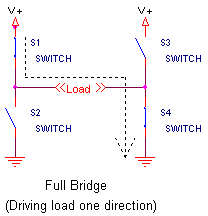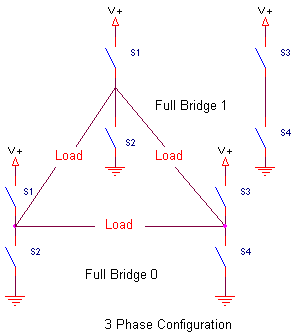# KMotion's Power Amplifiers and PWMs

The basic component of KMotion's switching power amplifiers is the Half Bridge (see below). A half bridge allows one point to be connected to either Motor Power (V +) or ground by ether closing switch S1 or S2 (but never both at the same time). The 4 Axis KMotion board contains a total of sixteen half bridges.To control a simple 2 point load (such as one of the two coils of a stepper motor) two half bridges are connected into what is called a Full Bridge (or sometimes referred to as an H Bridge).By closing specific switches, voltage may be applied either direction to the load or the load may be shorted (which applies 0 volts across the load) and may allow current to recirculate through the load. See the 3 diagrams below. This technique is what allows KMotion to apply positive voltage, negative voltage, or zero voltage to a particular load using only a single positive supply. Furthermore, in order to provide a variable voltage to a load, a method of PWM (Pulse Width Modulation) is utilized which rapidly (@ ~30KHz) switches between two of the three states. By varying the amount of time spent in the various states the average voltage that is applied to the load may be controlled.

There are two possible methods of performing PWM. KMotion refers to the two methods as Normal (in some literature this is referred to as locked anti-phase) or Recirculating.

The Normal method always switches between the first two states shown below (positive and negative). If the desired average voltage is positive, then the positive state will be applied for a longer time than the negative state. If the desired average voltage is negative, then the positive state will be applied for a shorter time than the negative state. For example, if +50% of the supply voltage is desired, then 75% of the time positive will be applied, and 25% of the time negative will be applied. Note that if the desired average voltage is zero, then the positive state will be applied for an equal time as the negative state. The advantage of this method is that the controlled voltage is very linear through zero. The slight disadvantage of this method is somewhat larger current ripple. Current ripple also becomes larger as the load inductance decreases. Most motors have relatively large inductances so this method works well and provides the best performance. However for loads with little or no inductance (such as a light bulb) this method is inappropriate as full current will always flow regardless of the +/- duty cycle.

The Recirculating method always switches between the zero state and either the positive state or the negative state depending on whether the desired voltage is either positive or negative. For example, if +50% of the supply voltage is desired, then 50% of the time positive will be applied, and 50% of the time zero will be applied. Note that if the desired average voltage is zero, then the zero state will be applied 100% of the time. This might be considered more of a true "off" condition, than the above method which switches equally between positive and negative. The advantage of this method is that there is less current ripple and will even work with non inductive loads (like a light bulb). The disadvantage of this method is the slight nonlinearity through zero that occurs when transitioning from a very short positive pulse to a very short negative pulse. Every real switch has a minimum turn on and turn off period which limits the smallest size of a pulse.KMotion also has a 3 Phase mode where two full bridges (only 1/2 of the second is used) and two PWM timers work in a special configuration in order to drive a 3 phase brushless motor. See the circuit configuration below. Here the supply voltage may be effectively applied at any phase angle (direction toward any of the 3 loads). The method utilized is analogous to the Normal mode described above and has smooth transitions through reversals.